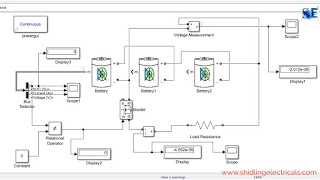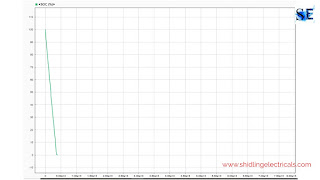# Lithium-Ion Battery Pack Discharging Circuit Matlab Simulink Simulation

Hello guys, welcome back to my blog. In this article, I will discuss the lithium-ion battery pack discharging circuit, Matlab Simulink simulation of the lithium-ion battery pack, its working, waveforms, etc.

If you need an article on some other topics then comment us below in the comment box. You can also catch me on Instagram - Chetan Shidling.

If you want to gain more knowledge about electrical and electronics, then visit www.cselectricalandelectronics.com

## Lithium-Ion Battery Pack Discharging Circuit Matlab Simulink Simulation

In this article, I will show a lithium-ion battery pack of 12 volts and 2600mAh. I will do a simulation in Matlab Simulink and I will give an explanation of each component, it's working, and waveforms such as SOC(state of charge), current, and voltage.Before Discharging

This is a discharging circuit of the lithium-ion battery pack which consists of three lithium-ion cells, load resistance, MOSFET, relational operator, constant, display, current measurement, voltage measurement, etc.

The three battery or cells are connected in series with the load resistance. When the cells are connected in series the voltage of each cell will be added. The nominal voltage of each cell is 3.7 and thus the total voltage of the battery pack is vary between 11 to 12 volts.

It takes 4000 seconds to discharge the lithium-ion battery pack completely to zero SOC. The below circuit shows the current and voltage of the battery pack.After discharging

### Components used:

#### 01. Lithium battery

The lithium-ion battery is nowadays very popular in electric vehicles. The nominal voltage of the lithium-ion cell is 3.7 volts, rated capacity is 2.6Ah, and the initial state of charge is 100%.

#### 02. Bus selector

By using a bus selector we can find SOC(state of charge), Current and voltage of the lithium-ion battery.

#### 03. Mosfet

It is used as a switch to open the circuit when the state of charge of the cell becomes zero. By using a relational operator we are controlling the switch.

#### 04. Relational operator

The relational operator is used to compare the state of charge and constant, if the state of charge is not equal to zero, the output of relational operator will be one(high) which makes MOSFET turn on. when the state of charge is equal to zero, the output of the relational operator will be zero(low) which makes MOSFET turn off.

#### 05. Constant

The constant block supplies the constant value to the relational operator.

#### 06. Display

By using the display block, we can display values of voltage, current, and state of charge.

#### 07. Current measurement

It is used to measure the value of the current. It is nothing but an ammeter in Matlab Simulink.

#### 08. Voltage measurement

The voltage measurement in Matlab Simulink is used to measure the voltage across the battery pack.

#### 09. Scope

The scope is used to display waveforms such as current, voltage, and current waveforms.

The value resistance I give is 4.4 ohms.

Working of lithium-ion battery pack discharge circuit

The total voltage of the battery pack is 11.6 volts, the current capacity is 2.6 Ah and the initial state of charge is 96%. When the MOSFET is turned on the current flow through load resistance. As the state of charge becomes zero, the MOSFET turns off and the circuit becomes open.

Now I will show you waveforms of currents, voltage, and state of charge.State of charge

State of charge waveform of the battery pack. The initial state of charge was 96% and when the load is connected the state of charge comes to zero.Current waveformVoltage waveform